# Ruby: Project Euler Problem # 97

http://projecteuler.net/problem=97

Large non-Mersenne prime

The first known prime found to exceed one million digits was discovered in 1999, and is a Mersenne prime of the form 269725931; it contains exactly 2,098,960 digits. Subsequently other Mersenne primes, of the form 2p1, have been found which contain more digits.
However, in 2004 there was found a massive non-Mersenne prime which contains 2,357,207 digits: 2843327830457+1.
Find the last ten digits of this prime number.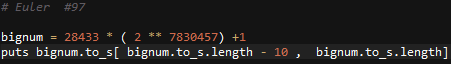# Ruby: Project Euler Problem # 58

http://projecteuler.net/problem=58

Spiral primes

Starting with 1 and spiralling anticlockwise in the following way, a square spiral with side length 7 is formed.

37 36 35 34 33 32 31
38 17 16 15 14 13 30
39 18  5  4  3 12 29
40 19  6  1  2 11 28
41 20  7  8  9 10 27
42 21 22 23 24 25 26
43 44 45 46 47 48 49

It is interesting to note that the odd squares lie along the bottom right diagonal, but what is more interesting is that 8 out of the 13 numbers lying along both diagonals are prime; that is, a ratio of 8/1362%.
If one complete new layer is wrapped around the spiral above, a square spiral with side length 9 will be formed. If this process is continued, what is the side length of the square spiral for which the ratio of primes along both diagonals first falls below 10%?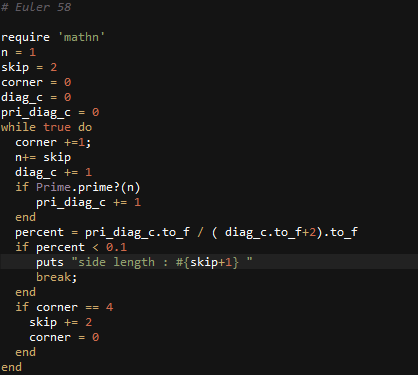# Ruby: Solution to Project Euler Problem # 53

http://projecteuler.net/problem=53

Combinatoric selections

There are exactly ten ways of selecting three from five, 12345:
123, 124, 125, 134, 135, 145, 234, 235, 245, and 345
In combinatorics, we use the notation, 5C3 = 10.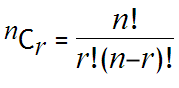where r n, n! = n(n1)…321, and 0! = 1.

How many, not necessarily distinct, values of  nCr, for 1n100, are greater than one-million?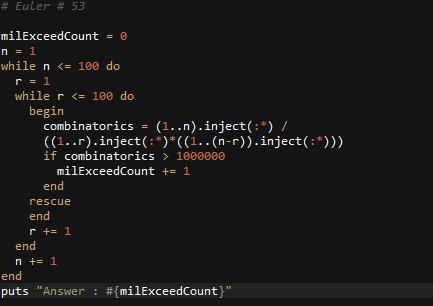# Ruby: Solution to Project Euler Problem # 56

http://projecteuler.net/problem=56

Powerful digit sum

A googol (10100) is a massive number: one followed by one-hundred zeros; 100100 is almost unimaginably large: one followed by two-hundred zeros. Despite their size, the sum of the digits in each number is only 1.

Considering natural numbers of the form, ab, where a, b100, what is the maximum digital sum?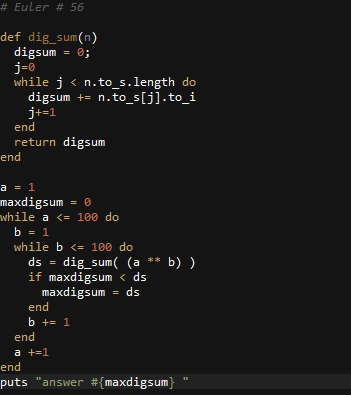# Ruby: Solution to Project Euler Problem # 28

http://projecteuler.net/problem=28

Number spiral diagonals

Starting with the number 1 and moving to the right in a clockwise direction a 5 by 5 spiral is formed as follows:

21 22 23 24 25
20  7  8  9 10
19  6  1  2 11
18  5  4  3 12
17 16 15 14 13

It can be verified that the sum of the numbers on the diagonals is 101.

What is the sum of the numbers on the diagonals in a 1001 by 1001 spiral formed in the same way?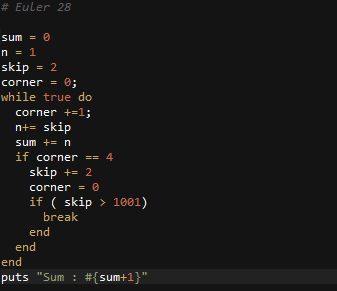# Ruby: Solution to Project Euler Problem # 40

http://projecteuler.net/problem=40

Champernowne’s constant

An irrational decimal fraction is created by concatenating the positive integers:

0.123456789101112131415161718192021…

If dn represents the nth digit of the fractional part, find the value of the following expression.

d1d10d100d1000d10000d100000d1000000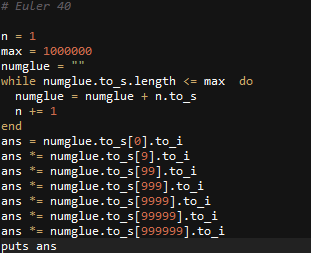# Ruby: Solution to Project Euler Problem # 25

Recently, I’ve been typing more Ruby then usual. In Ruby you can have a fairly big number.
http://projecteuler.net/problem=25

1000-digit Fibonacci number

The Fibonacci sequence is defined by the recurrence relation:

Fn = Fn1 + Fn2, where F1 = 1 and F2 = 1.

The 12th term, F12, is the first term to contain three digits.

What is the first term in the Fibonacci sequence to contain 1000 digits?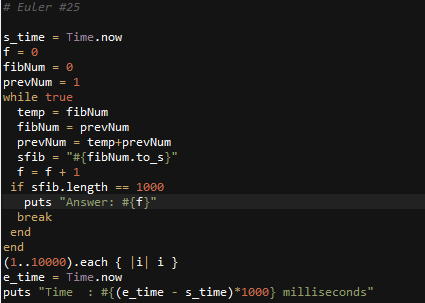# C#: Project Euler Solution to Problem 27

http://projecteuler.net/problem=27

```static void Main(string[] args) { //n² + an + b int maxprimes = 0; int maxproduct = 0; for (int a = 0; a < 1000; a++) { for (int b = 0; b < 1000; b++) { int prime = countPrime(a, b); if (maxprimes < prime) { maxprimes = prime; maxproduct= (-a*b ); } } } Console.WriteLine(maxproduct); }   public static int countPrime(int a, int b) { int count = 0; int n = 0; while (true) { double result = Math.Pow(n, 2.00) - (a * n) + b; if (isPrime((int)result) && result >= 0) count++; else return count; n++; } }   public static bool isPrime(int n) { if (n == 1) return false; if (n == 2) return true; for (int i = 2; i < n; ++i) { if ((n % i) == 0) return false; } return true; }```